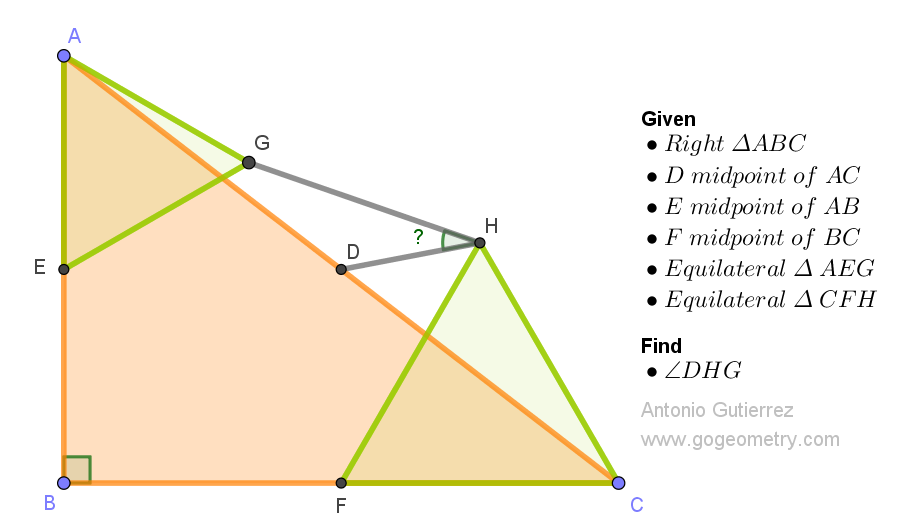# Geometry Problem 1557: Calculate the Angle DHG in Right Triangle ABC. The problem involves Equilateral, Isosceles, Midpoint and Congruence

In the right triangle ABC, with a right angle at B, points D, E, and F are located at the midpoints of the sides AC, AB, and BC, respectively. Then, the equilateral triangles AEG and CFH are constructed, as shown in the figure. Calculate the measure of angle DHG.In right-angled embrace,
Midpoints form triangles' grace,
Angles whisper tales,
Seeking DHG's hidden place,
Geometry's mystic trails.